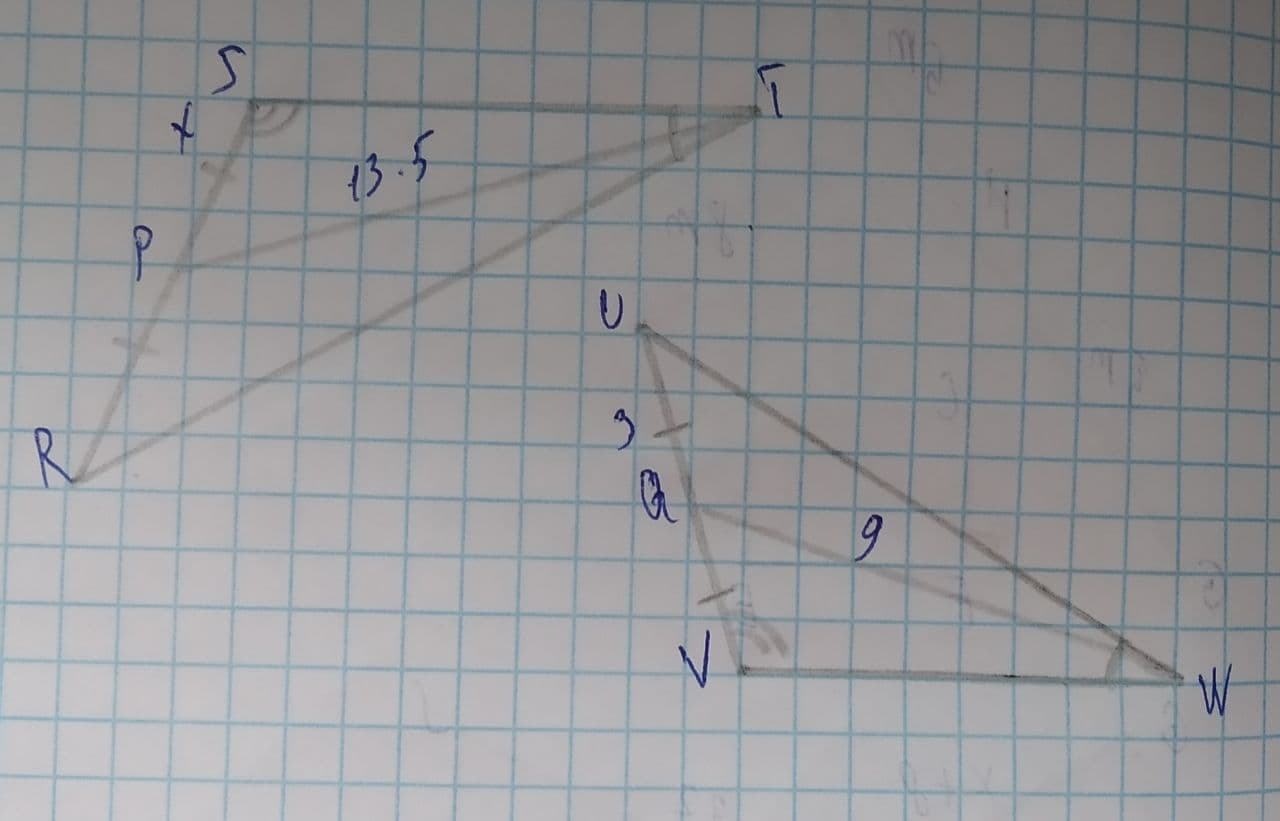# To find: The value of x.Tammy Todd 2021-08-11 Answered
To find: The value of x.
Given:• Questions are typically answered in as fast as 30 minutes

### Plainmath recommends

• Get a detailed answer even on the hardest topics.
• Ask an expert for a step-by-step guidance to learn to do it yourself.nitruraviX
In $$\displaystyle\triangle{S}{T}{R},\triangle{V}{W}{U}$$:
$$\displaystyle\angle{S}\stackrel{\sim}{=}\angle{V}$$
$$\displaystyle\angle{T}\stackrel{\sim}{=}\angle{W}$$
By A-A similarity, the $$\displaystyle\triangle{S}{T}{R}\sim\triangle{V}{W}{U}$$.
SP=PR=x
$$\displaystyle\Rightarrow{S}{R}={2}{x}$$
UQ=QV=3
$$\displaystyle\Rightarrow{U}{V}={6}$$
In two similar triangles, the ratio of their corresponding sides, medians are equal.
$$\displaystyle{\frac{{{S}{R}}}{{{V}{U}}}}={\frac{{{T}{P}}}{{{W}{Q}}}}$$
$$\displaystyle\Rightarrow{\frac{{{2}{x}}}{{{6}}}}={\frac{{{13.5}}}{{{9}}}}$$
$$\displaystyle\Rightarrow{x}={4.5}$$